SLVSC31D December   2013  – August 2015

PRODUCTION DATA.

1. Features
2. Applications
3. Description
4. Revision History
5. Description (continued)
6. Pin Configuration and Functions
7. Specifications
8. Detailed Description
1. 8.1 Overview
2. 8.2 Functional Block Diagram
3. 8.3 Feature Description
4. 8.4 Device Functional Modes
9. Application and Implementation
1. 9.1 Application Information
2. 9.2 Typical Application
10. 10Power Supply Recommendations
11. 11Layout
12. 12Device and Documentation Support
13. 13Mechanical, Packaging, and Orderable Information

• U|10
• KGD|0
• HKU|10

## 9 Application and Implementation

NOTE

Information in the following applications sections is not part of the TI component specification, and TI does not warrant its accuracy or completeness. TI’s customers are responsible for determining suitability of components for their purposes. Customers should validate and test their design implementation to confirm system functionality.

### 9.1 Application Information

The TPS7A4501-SP regulator has very-low output noise, which makes it ideal for sensitive RF supply applications.

### 9.2 Typical Application

This section highlights some of the design considerations when implementing this device in various applications.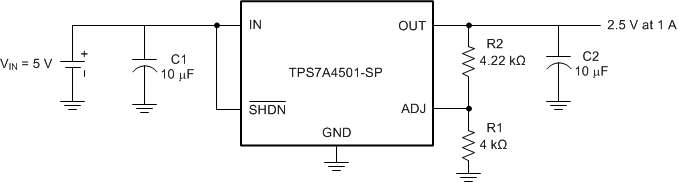Figure 23. Adjustable Output Voltage Operation

#### 9.2.1 Design Requirements

Table 2 shows the design requirements.

### Table 2. Design Parameters

DESIGN PARAMETER EXAMPLE VALUE
Input voltage (VIN) 5 V
Output voltage (VOUT) 2.5 V
Output current (IOUT) 0 to 1 A

#### 9.2.2 Detailed Design Procedure

The TPS7A4501-SP has an adjustable output voltage range of 1.21 to 20 V. The output voltage is set by the ratio of two external resistors, R1 and R2, as shown in Figure 23. The device maintains the voltage at the ADJ pin at 1.21 V referenced to ground. The current in R1 is then equal to (1.21 V/R1), and the current in R2 is the current in R1 plus the ADJ pin bias current. The ADJ pin bias current, 3 µA at 25°C, flows through R2 into the ADJ pin. Calculate the output voltage using Equation 2.

Equation 2.The value of R1 should be less than 4.17 kΩ to minimize errors in the output voltage caused by the ADJ pin bias current. Note that in shutdown the output is turned off, and the divider current is zero. For an output voltage of 2.50 V, R1 is set to 4 kΩ. R2 is then found to be 4.22 kΩ using Equation 2.

Equation 3.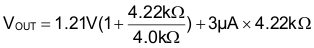Equation 4. VOUT = 2.50 V

The adjustable device is tested and specified with the ADJ pin tied to the OUT pin for an output voltage of 1.21 V. Specifications for output voltages greater than 1.21 V are proportional to the ratio of the desired output voltage to 1.21 V: VOUT / 1.21 V. For example, load regulation for an output current change of 1 mA to 1.5 A is –2 mV (typical) at VOUT = 1.21 V. At VOUT = 2.50 V, the typical load regulation is:

Equation 5. (2.50 V / 1.21 V)(–2 mV) = –4.13 mV

shows the actual change in output is about 3 mV for a 1-A load step. The maximum load regulation at 25°C is –8 mV. At VOUT = 2.50 V, the maximum load regulation is:

Equation 6. (2.50 V / 1.21 V)(–8 mV) = –16.53 mV

Because 16.53 mV is only 0.7% of the 2.5-V output voltage, the load regulation meets the design requirements.

#### 9.2.2.1 Output Capacitance and Transient Response

The TPS7A4501-SP regulator is designed to be stable with a wide range of output capacitors. The ESR of the output capacitor affects stability, most notably with small capacitors. TI recommends a minimum output capacitor of 10 μF with an ESR of 3 Ω or less to prevent oscillations. Larger values of output capacitance can decrease the peak deviations and provide improved transient response for larger load current changes. Bypass capacitors, used to decouple individual components powered by the TPS7A4501-SP, increase the effective output capacitor value.

Give extra consideration to the use of ceramic capacitors. Ceramic capacitors are manufactured with a variety of dielectrics, each with different behavior over temperature and applied voltage. The most common dielectric used for a harsh environment is X7R. Ceramic capacitors lose capacitance when DC bias is applied across the capacitor. This capacitance loss is due to the polarization of the ceramic material. The capacitance loss is not permanent: after a large DC bias is applied, reducing the DC bias reduces the degree of polarization and capacitance increases. DC bias effects vary dramatically with voltage rating, case size, capacitor value, and capacitor manufacturer. Because a capacitor could lose more than 50% of its capacitance with DC bias voltages near the voltage rating of the capacitor, it is important to consider DC bias when selecting a ceramic capacitor for an application.

Ceramic capacitors' dielectric also changes over the temperature range. For example X7R, the first letter X denotes lower temperature range –55°C whereas 7 denotes a higher temperature range 125°C and R denotes capacitance variation over the temperature range (±15%). For harsh environment applications, minimum dielectric thickness must be 1 mil for 100-V DC-rated capacitor and 0.8 mil for 50-V DC-rated capacitors.

Voltage and temperature coefficients are not the only sources of problems. Some ceramic capacitors have a piezoelectric response. A piezoelectric device generates voltage across its terminals due to mechanical stress, similar to the way a piezoelectric accelerometer or microphone works. For a ceramic capacitor, the stress can be induced by vibrations in the system or thermal transients.

Tantalum capacitors can provide higher capacitance per unit volume. Tantalum capacitors can be either manganese dioxide (MNO2)-based capacitors where the cathode is MN02 or polymer. MN02-based tantalum capacitors exhibit high ESR as compared to polymer-based tantalum capacitors. MN02-based tantalum capacitors require in excess of 60% voltage derating. Thus, a 10-V rated capacitor can only be used for 3.3-V application. Whereas polymer-based capacitors only require 10% voltage derating. Paralleling ceramic and tantalum capacitors provide optimum balance between capacitance and ESR.

Table 3 highlights some of the capacitors used in the device.

### Table 3. TPS7A4501-SP Capacitors

CAPACITOR PART NUMBER CAPACITOR DETAILS TYPE VENDOR
(CAPACITOR, VOLTAGE, ESR)
TYPE VENDOR
T493X226M025AH6x20 22 μF, 25 V, 35 mΩ Tantalum - MnO2 Kemet
T525D476M016ATE035 47 μF, 10 V, 35 mΩ Tantalum - Polymer Kemet
T525D107M010ATE025 100 μF, 10 V, 25 mΩ Tantalum - Polymer Kemet
T541X337M010AH6720 330 μF, 10 V, 6 mΩ Tantalum - Polymer Kemet
T525D227M010ATE025 220 μF, 10 V, 25 mΩ Tantalum - Polymer Kemet
T495X107K016ATE100 100 μF, 16 V, 100 mΩ Tantalum - MnO2 Kemet
CWR29FK227JTHC 220 μF, 10 V, 180 mΩ Tantalum - MnO2 AVX
THJE107K016AJH 100 μF, 16 V, 58 mΩ Tantalum AVX
THJE227K010AJH 220 μF, 10 V, 40 mΩ Tantalum AVX
SR2225X7R335K1P5#M123 3.3 μF, 25 V, 10 mΩ Ceramic Presidio Components Inc

#### 9.2.2.2 Compensation

TPS7A4501-SP is internally compensated. However, the user can implement a lead network using C3 to boost the phase margin as well as reduce output noise.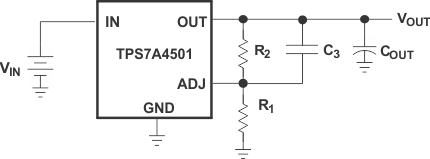Figure 24. Compensation Schematic

R1, the bottom resistor, and R2, the top resistor, form the output voltage divider network. C3 across R2 adds a lead network.

For R1 = 3.2 kΩ and R2 = 10 kΩ, VOUT is set at 5 V and C3 = 470 pF.

Zero and pole can be calculated as shown in the following equations.

Equation 7.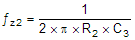Equation 8. ƒz2 = 33.863 kHz
Equation 9.Equation 10. R1p = 2.424 kΩ
Equation 11.Equation 12. ƒp2 = 139.684 kHz

#### 9.2.3 Application Curves

The following waveforms indicate the transient behavior of the TPS7A4501-SP.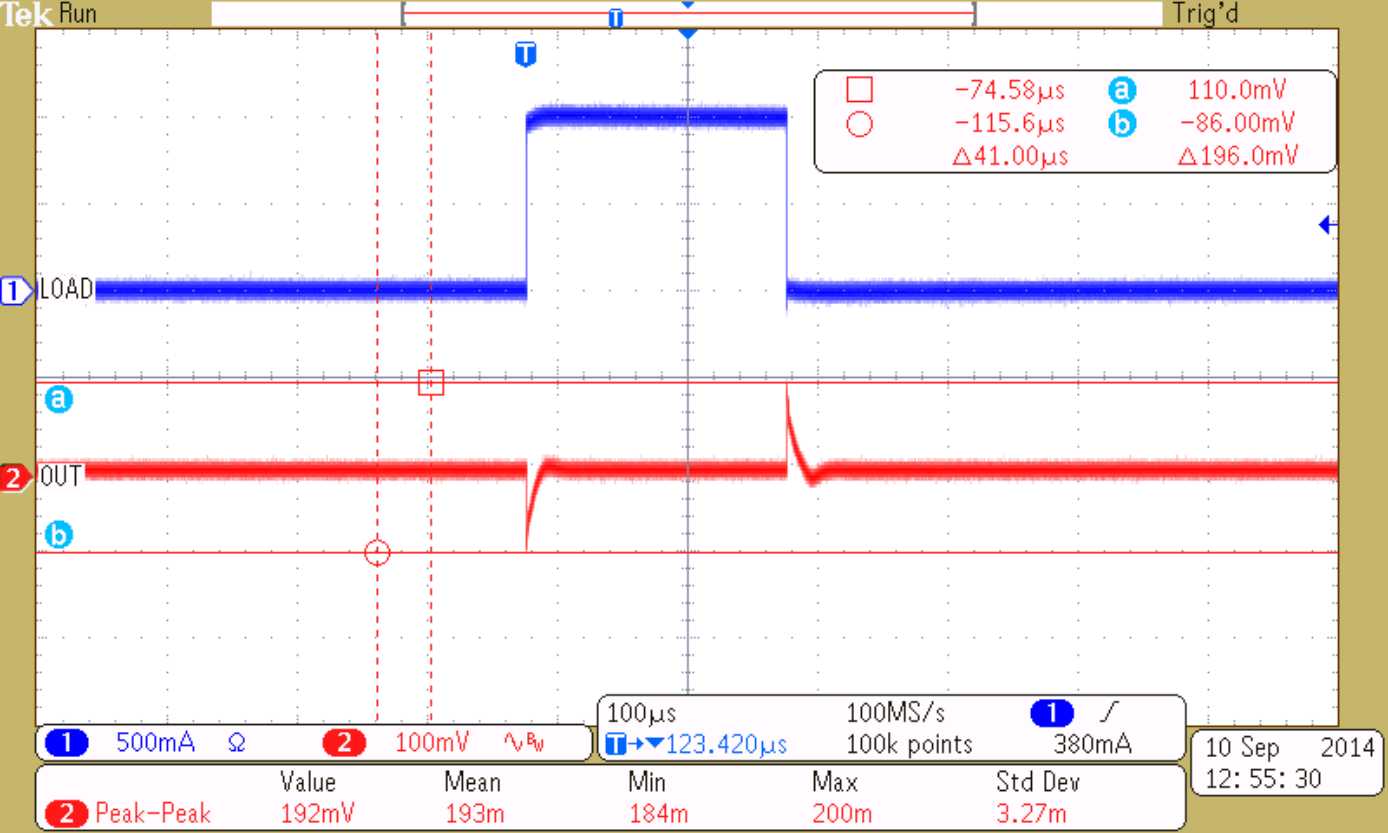Figure 25. 1-A Load Transient Response, Step Load is from 0.1 to 1.1 A, Vin = 5 V, Vout = 2.5 V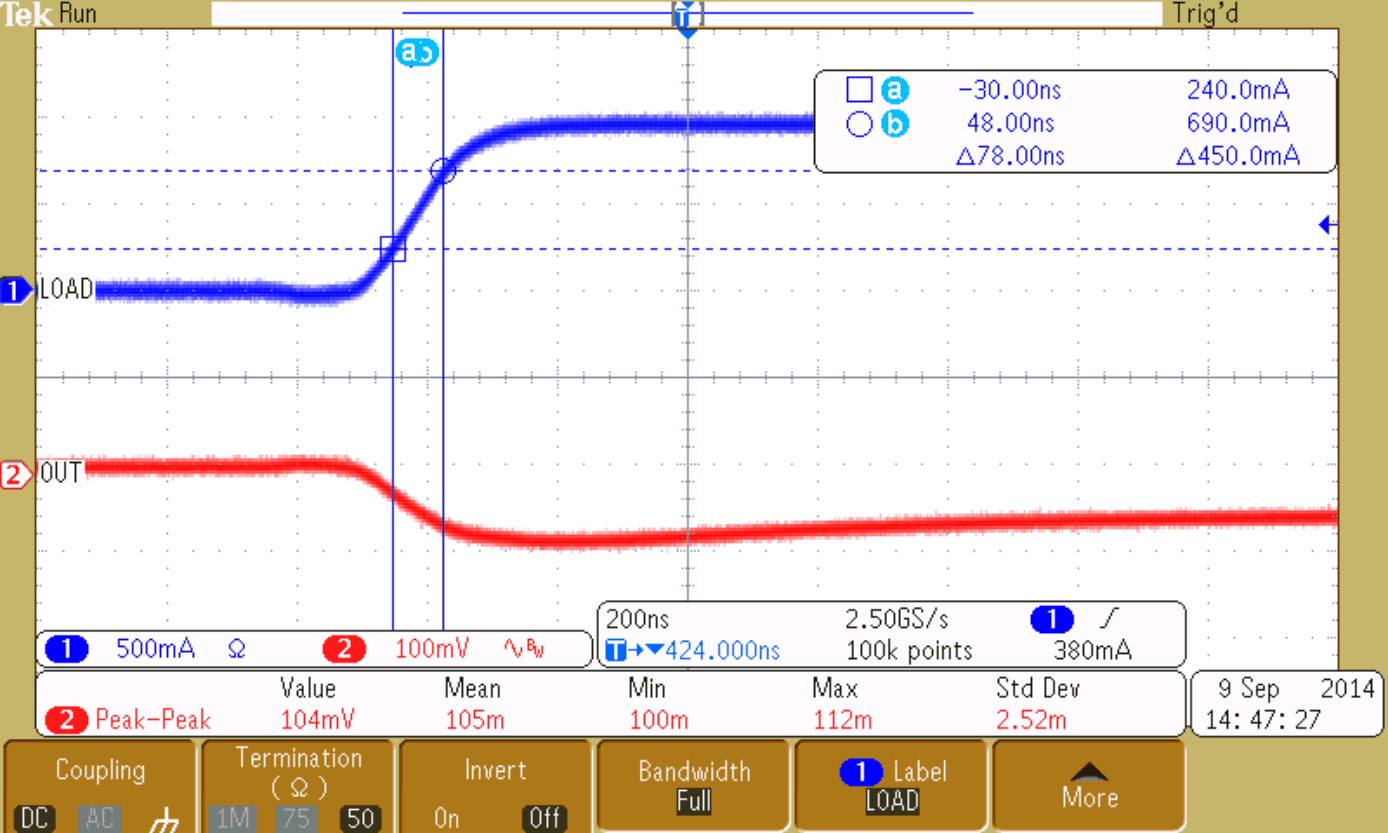Figure 27. 1-A Load, Expanded View (Low to High), Slew Rate = 5.8 A/μS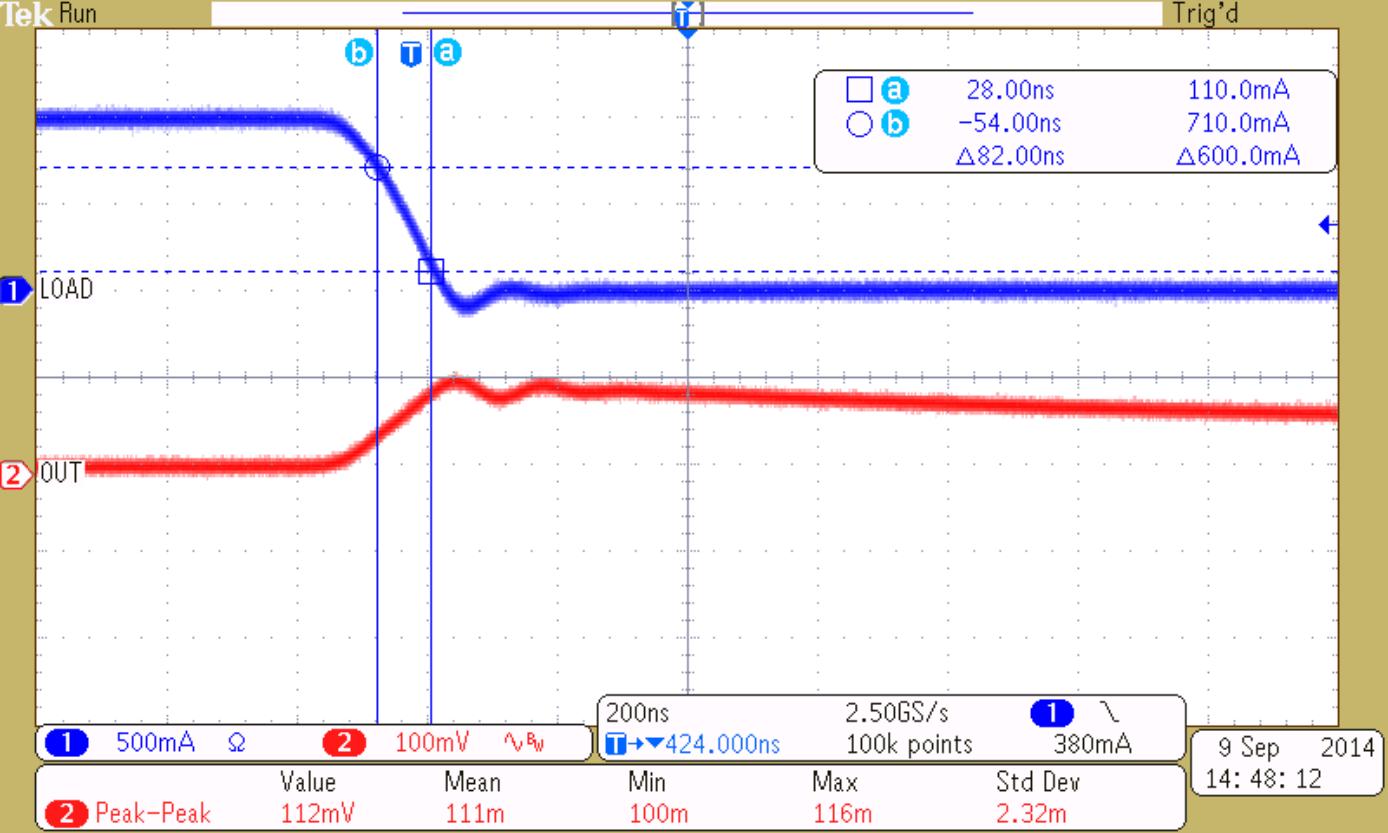Figure 26. 1-A Load, Expanded View (High to Low), Slew Rate = 7.3 A/μS